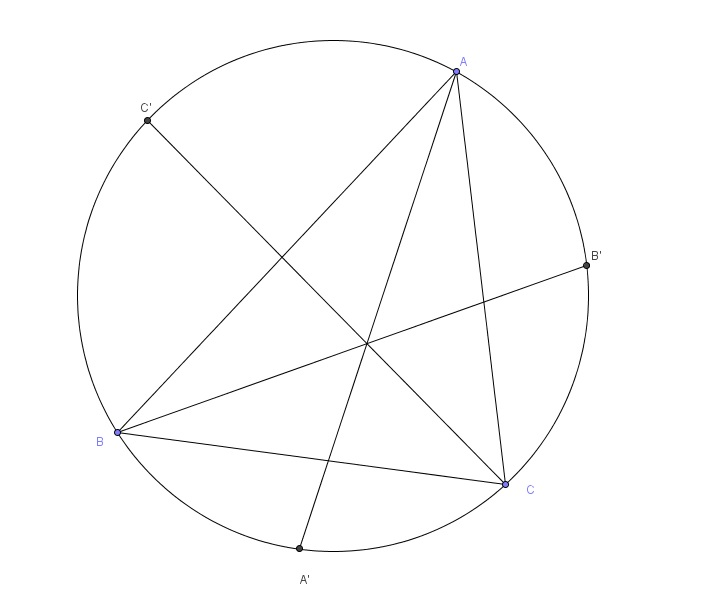# Extended Bisector

Geometry Level 3Triangle $ABC$ is inscribed in a unit circle. The three bisectors of the angle $A$, $B$ and $C$ are extended to intersect the circle at $A'$, $B'$ and $C'$ respectively. Determine the value of

$\frac{AA'\cos\frac{A}{2}+BB'\cos\frac{B}{2}+CC'\cos\frac{C}{2}}{\sin A+\sin B+\sin C}$

×